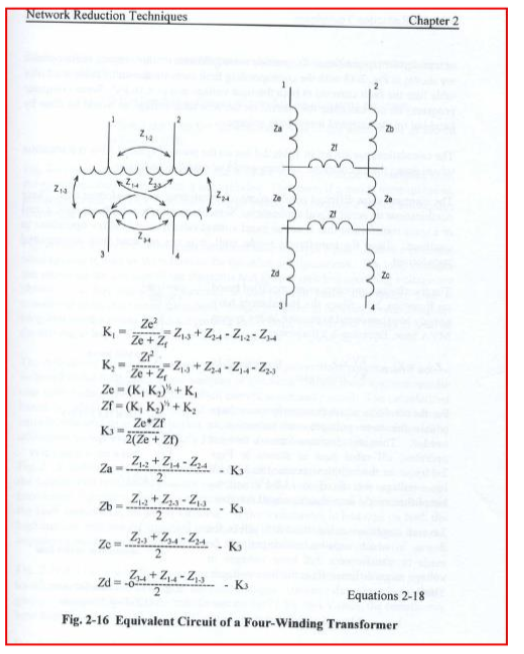• Sales
• Library Model Request
• Technical Support

# Modeling 4-Winding Transformer

How do I model Four Winding Transformer in PTW?

Solution:
Using as reference "A Practical Guide to Short-Circuit Calculation" by Conrad St. Pierre, Page 37, users can model four-winding transformer as an 8 buses system.Four winding Transformer model can be modeled as an 8-bus system.

Example 1
To make things simple, assume Z13=Z24=Z12=Z34=Z14=Z23=6.5%. This means that the transformer is

This model can be translated into:

K1= Z13+Z24-Z12-Z34;
K2= Z13+Z24-Z14-Z23;
Ze = sqrt(K1*K2) + K1;
Zf = sqrt(K1*K2) + K2;
if(abs(Ze+Zf) < 0.00001)
K3 = 0.;
else
K3=Ze*Zf/2./(Ze+Zf);
Za=(Z12+Z14-Z24)/2. -K3;
Zb=(Z12+Z23-Z13)/2. -K3;
Zc=(Z23+Z34-Z24)/2. -K3;
Zd=(Z34+Z14-Z13)/2. -K3;

Given:
Z13=Z24=Z12=Z34=6.5%

Results:
K1=K2=Ze=Zf=0.

Za=Zb=Zc=Zd= 3.25%